# Image Compression using Huffman Coding

Huffman coding is one of the basic compression methods, that have proven useful in image and video compression standards. When applying Huffman encoding technique on an Image, the source symbols can be either pixel intensities of the Image, or the output of an intensity mapping function.

Prerequisites : Huffman Coding | File Handling

The first step of Huffman coding technique is to reduce the input image to a ordered histogram, where the probability of occurrence of a certain pixel intensity value is as

 prob_pixel = numpix/totalnum

where numpix is the number of occurrence of a pixel with a certain intensity value and totalnum is the total number of pixels in the input Image.

Let us take a 8 X 8 Image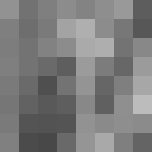The pixel intensity values are :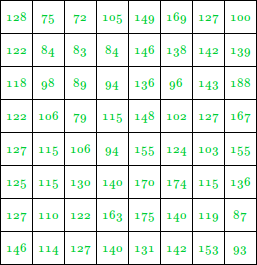This image contains 46 distinct pixel intensity values, hence we will have 46 unique Huffman code words.

It is evident that, not all pixel intensity values may be present in the image and hence will not have non-zero probability of occurrence.

From here on, the pixel intensity values in the input Image will be addressed as leaf nodes.

Now, there are 2 essential steps to build a Huffman Tree :

1. Build a Huffman Tree :
1. Combine the two lowest probability leaf nodes into a new node.
2. Replace the two leaf nodes by the new node and sort the nodes according to the new probability values.
3. Continue the steps (a) and (b) until we get a single node with probability value 1.0. We will call this node as root
2. Backtrack from the root, assigning ‘0’ or ‘1’ to each intermediate node, till we reach the leaf nodes

In this example, we will assign ‘0’ to the left child node and ‘1’ to the right one.

Now, let’s look into the implementation :
Step 1 :
Read the Image into a 2D array(image)
If the Image is in .bmp format, then the Image can be read into the 2D array, by using this code given in this link here.

 int i, j;  char filename[] = "Input_Image.bmp";  int data = 0, offset, bpp = 0, width, height;  long bmpsize = 0, bmpdataoff = 0;  int** image;  int temp = 0;      // Reading the BMP File  FILE* image_file;  image_file = fopen(filename, "rb");  if (image_file == NULL)  {      printf("Error Opening File!!");      exit(1);  }  else  {         // Set file position of the       // stream to the beginning      // Contains file signature       // or ID "BM"      offset = 0;             // Set offset to 2, which       // contains size of BMP File      offset = 2;             fseek(image_file, offset, SEEK_SET);             // Getting size of BMP File      fread(&bmpsize, 4, 1, image_file);             // Getting offset where the      // pixel array starts      // Since the information       // is at offset 10 from       // the start, as given       // in BMP Header      offset = 10;              fseek(image_file, offset, SEEK_SET);             // Bitmap data offset      fread(&bmpdataoff, 4, 1, image_file);             // Getting height and width of the image      // Width is stored at offset 18 and height      // at offset 22, each of 4 bytes      fseek(image_file, 18, SEEK_SET);             fread(&width, 4, 1, image_file);             fread(&height, 4, 1, image_file);             // Number of bits per pixel      fseek(image_file, 2, SEEK_CUR);             fread(&bpp, 2, 1, image_file);             // Setting offset to start of pixel data      fseek(image_file, bmpdataoff, SEEK_SET);             // Creating Image array      image = (int**)malloc(height * sizeof(int*));      for (i = 0; i < height; i++)      {          image[i] = (int*)malloc(width * sizeof(int));      }             // int image[height][width]       // can also be done      // Number of bytes in the       // Image pixel array      int numbytes = (bmpsize - bmpdataoff) / 3;              // Reading the BMP File       // into Image Array      for (i = 0; i < height; i++)       {          for (j = 0; j < width; j++)          {              fread(&temp, 3, 1, image_file);                             // the Image is a               // 24-bit BMP Image              temp = temp & 0x0000FF;               image[i][j] = temp;          }      }  }

Create a Histogram of the pixel intensity values present in the Image

 // Creating the Histogram  int hist;     for (i = 0; i < 256; i++)      hist[i] = 0;     for (i = 0; i < height; i++)      for (j = 0; j < width; j++)          hist[image[i][j]] += 1;

Find the number of pixel intensity values having non-zero probability of occurrence
Since, the values of pixel intensities range from 0 to 255, and not all pixel intensity values may be present in the image (as evident from the histogram and also the image matrix) and hence will not have non-zero probability of occurrence. Also another purpose this step serves, is that the number of pixel intensity values having non-zero probability values will give us the number of leaf nodes in the Image.

 // Finding number of   // non-zero occurrences  int nodes = 0;  for (i = 0; i < 256; i++)   {      if (hist[i] != 0)          nodes += 1;  }

Calculating the maximum length of Huffman code words
As shown by Y.S.Abu-Mostafa and R.J.McEliece in their paper “Maximal codeword lengths in Huffman codes”, that, If, then in any efficient prefix code for a source whose least probability is p, the longest codeword length is at most K & If, there exists a source whose smallest probability is p, and which has a Huffman code whose longest word has length K. If, there exists such a source for which every optimal code has a longest word of length K.
Here,is theFibonacci number.

Gallager  noted that every Huffman tree is efficient, but in fact it is easy to see more
generally that every optimal tree is efficient

Fibonacci Series is : 0, 1, 1, 2, 3, 5, 8, 13, 21, 34, 55, 89, 144, …

In our example, lowest probability(p) is 0.015625

Hence,

 1/p = 64
For K = 9,
F(K+2) = F(11) = 55
F(K+3) = F(12) = 89

Therefore,

1/F(K+3) < p < 1/F(K+2)
Hence optimal length of code is K=9

 // Calculating max length   // of Huffman code word  i = 0;  while ((1 / p) < fib(i))      i++;  int maxcodelen = i - 3;

 // Function for getting Fibonacci  // numbers defined outside main  int fib(int n)  {      if (n <= 1)          return n;      return fib(n - 1) + fib(n - 2);  }

Step 2
Define a struct which will contain the pixel intensity values(pix), their corresponding probabilities(freq), the pointer to the left(*left) and right(*right) child nodes and also the string array for the Huffman code word(code).

These structs is defined inside main(), so as to use the maximum length of code(maxcodelen) to declare the code array field of the struct pixfreq

 // Defining Structures pixfreq  struct pixfreq   {      int pix;      float freq;      struct pixfreq *left, *right;      char code[maxcodelen];  };

Step 3
Define another Struct which will contain the pixel intensity values(pix), their corresponding probabilities(freq) and an additional field, which will be used for storing the position of new generated nodes(arrloc).

 // Defining Structures huffcode  struct huffcode   {      int pix, arrloc;      float freq;  };

Step 4
Declaring an array of structs. Each element of the array corresponds to a node in the Huffman Tree.

 // Declaring structs  struct pixfreq* pix_freq;  struct huffcode* huffcodes;

Why use two struct arrays?

Initially, the struct array pix_freq, as well as the struct array huffcodes will only contain the information of all the leaf nodes in the Huffman Tree.

The struct array pix_freq will be used to store all the nodes of the Huffman Tree and the array huffcodes will be used as the updated (and sorted) tree.

Remember that, only huffcodes will be sorted in each iteration, and not pix_freq

The new nodes created by combining two nodes of lowest frequency, in each iteration, will be appended to the end of the pix_freq array, and also to huffcodes array.

But the array huffcodes will be sorted again according to the probability of occurrence, after the new node is added to it.

The position of the new node in the array pix_freq will be stored in the arrloc field of the struct huffcode.
The arrloc field will be used when assigning the pointer to the left and right child of a new node.

Step 4 continued…

Now, if there are N number of leaf nodes, the total number of nodes in the whole Huffman Tree will be equal to 2N-1

And after two nodes are combined and replaced by the new parent node, the number of nodes decreases by 1 at each iteration. Hence, it is sufficient to have a length of nodes for the array huffcodes, which will be used as the updated and sorted Huffman nodes.

 int totalnodes = 2 * nodes - 1;  pix_freq = (struct pixfreq*)malloc(sizeof(struct pixfreq) * totalnodes);  huffcodes = (struct huffcode*)malloc(sizeof(struct huffcode) * nodes);

Step 5
Initialize the two arrays pix_freq and huffcodes with information of the leaf nodes.

 j = 0;  int totpix = height * width;  float tempprob;  for (i = 0; i < 256; i++)   {      if (hist[i] != 0)      {                     // pixel intensity value          huffcodes[j].pix = i;           pix_freq[j].pix = i;                     // location of the node          // in the pix_freq array          huffcodes[j].arrloc = j;                      // probability of occurrence          tempprob = (float)hist[i] / (float)totpix;           pix_freq[j].freq = tempprob;          huffcodes[j].freq = tempprob;                     // Declaring the child of           // leaf node as NULL pointer          pix_freq[j].left = NULL;           pix_freq[j].right = NULL;                     // initializing the code          // word as end of line          pix_freq[j].code = '\0';           j++;      }  }

Step 6
Sorting the huffcodes array according to the probability of occurrence of the pixel intensity values

Note that, it is necessary to sort the huffcodes array, but not the pix_freq array, since we are already storing the location of the pixel values in the arrloc field of the huffcodes array.

 // Sorting the histogram  struct huffcode temphuff;     // Sorting w.r.t probability   // of occurrence  for (i = 0; i < nodes; i++)   {      for (j = i + 1; j < nodes; j++)   {          if (huffcodes[i].freq < huffcodes[j].freq)   {              temphuff = huffcodes[i];              huffcodes[i] = huffcodes[j];              huffcodes[j] = temphuff;          }      }  }

Step 7
Building the Huffman Tree

We start by combining the two nodes with lowest probabilities of occurrence and then replacing the two nodes by the new node. This process continues until we have a root node. The first parent node formed will be stored at index nodes in the array pix_freq and the subsequent parent nodes obtained will be stored at higher values of index.

 // Building Huffman Tree     float sumprob;  int sumpix;  int n = 0, k = 0;  int nextnode = nodes;     // Since total number of   // nodes in Huffman Tree   // is 2*nodes-1  while (n < nodes - 1)   {             // Adding the lowest       // two probabilities      sumprob = huffcodes[nodes - n - 1].freq + huffcodes[nodes - n - 2].freq;      sumpix = huffcodes[nodes - n - 1].pix + huffcodes[nodes - n - 2].pix;             // Appending to the pix_freq Array      pix_freq[nextnode].pix = sumpix;      pix_freq[nextnode].freq = sumprob;      pix_freq[nextnode].left = &pix_freq[huffcodes[nodes - n - 2].arrloc];             // arrloc points to the location      // of the child node in the      // pix_freq array      pix_freq[nextnode].right = &pix_freq[huffcodes[nodes - n - 1].arrloc];      pix_freq[nextnode].code = '\0';             // Using sum of the pixel values as       // new representation for the new node      // since unlike strings, we cannot       // concatenate because the pixel values       // are stored as integers. However, if we      // store the pixel values as strings      // we can use the concatenated string as       // a representation of the new node.      i = 0;             // Sorting and Updating the huffcodes       // array simultaneously New position      // of the combined node      while (sumprob <= huffcodes[i].freq)          i++;                 // Inserting the new node in      // the huffcodes array      for (k = nnz; k >= 0; k--)       {          if (k == i)          {              huffcodes[k].pix = sumpix;              huffcodes[k].freq = sumprob;              huffcodes[k].arrloc = nextnode;          }          else if (k > i)                         // Shifting the nodes below              // the new node by 1              // For inserting the new node               // at the updated position k              huffcodes[k] = huffcodes[k - 1];      }      n += 1;      nextnode += 1;  }

How does this code work?

Let’s see that by an example:

Initially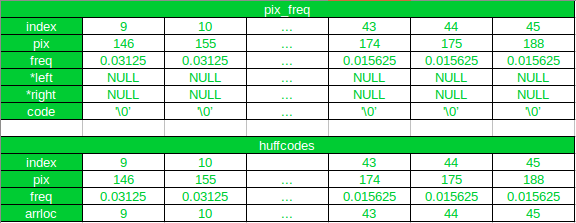After the First Iteration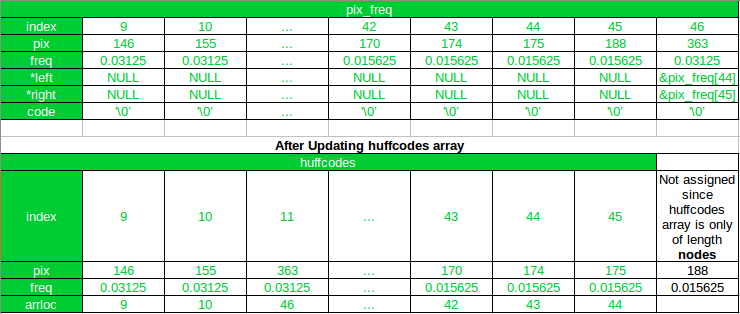As you can see, after first iteration, the new node has been appended to the pix_freq array, and it’s index is 46. And in the huffcode the new node has been added at its new position after sorting, and the arrloc points to the index of the new node in the pix_freq array. Also, notice that, all array elements after the new node (at index 11) in huffcodes array has been shifted by 1 and the array element with pixel value 188 gets excluded in the updated array.

Now, in the next(2nd) iteration 170 and 174 will be combined, since 175 and 188 has already been combined.
Index of the lowest two nodes in terms of the variable nodes and n is

left_child_index=(nodes-n-2)

and

right_child_index=(nodes-n-1)

In 2nd iteration, value of n is 1 (since n starts from 0).

For node having value 170

 left_child_index=46-1-2=43

For node having value 174

 right_child_index=46-1-1=44

Hence, even if 175 remains the last element of the updated array, it will get excluded.

Another thing to notice in this code, is that, if in any subsequent iteration, the new node formed in the first iteration is the child of another new node, then the pointer to the new node obtained in the first iteration, can be accessed using the arrloc stored in huffcodes array, as is done in this line of code

 pix_freq[nextnode].right = &pix_freq[huffcodes[nodes - n - 1].arrloc];

Step 8
Backtrack from the root to the leaf nodes to assign code words

Starting from the root, we assign ‘0’ to the left child node and ‘1’ to the right child node.

Now, since we were appending the newly formed nodes to the array pix_freq, hence it is expected that the root will be the last element of the array at index totalnodes-1.

Hence, we start from the last index and iterate over the array, assigning code words to the left and right child nodes, till we reach the first parent node formed at index nodes. We don’t iterate over the leaf nodes since those nodes has NULL pointers as their left and right child.

 // Assigning Code through backtracking  char left = '0';  char right = '1';  int index;  for (i = totalnodes - 1; i >= nodes; i--) {      if (pix_freq[i].left != NULL) {          strconcat(pix_freq[i].left->code, pix_freq[i].code, left);      }      if (pix_freq[i].right != NULL) {          strconcat(pix_freq[i].right->code, pix_freq[i].code, right);      }  }

 void strconcat(char* str, char* parentcode, char add)  {      int i = 0;      while (*(parentcode + i) != '\0') {          *(str + i) = *(parentcode + i);          i++;      }      str[i] = add;      str[i + 1] = '\0';  }

Final Step
Encode the Image

 // Encode the Image  int pix_val;     // Writing the Huffman encoded  //  Image into a text file  FILE* imagehuff = fopen("encoded_image.txt", "wb");  for (r = 0; r < height; r++)      for (c = 0; c < width; c++) {          pix_val = image[r];          for (i = 0; i < nodes; i++)              if (pix_val == pix_freq[i].pix)                  fprintf(imagehuff, "%s", pix_freq[i].code);      }  fclose(imagehuff);     // Printing Huffman Codes  printf("Huffmann Codes::\n\n");  printf("pixel values ->   Code\n\n");  for (i = 0; i < nodes; i++) {      if (snprintf(NULL, 0, "%d", pix_freq[i].pix) == 2)          printf("     %d      -> %s\n", pix_freq[i].pix, pix_freq[i].code);      else         printf("    %d      -> %s\n", pix_freq[i].pix, pix_freq[i].code);  }

Another important point to note
Average number of bits required to represent each pixel.

 // Calculating Average number of bits  float avgbitnum = 0;  for (i = 0; i < nodes; i++)      avgbitnum += pix_freq[i].freq * codelen(pix_freq[i].code);

The function codelen calculates the length of codewords OR, the number of bits required to represent the pixel.

 int codelen(char* code)  {      int l = 0;      while (*(code + l) != '\0')          l++;      return l;  }

For this specific example image

Average number of bits = 5.343750

The printed results for the example image

    pixel values -> Code
72      -> 011001
75      -> 010100
79      -> 110111
83      -> 011010
84      -> 00100
87      -> 011100
89      -> 010000
93      -> 010111
94      -> 00011
96      -> 101010
98      -> 101110
100      -> 000101
102      -> 0001000
103      -> 0001001
105      -> 110110
106      -> 00110
110      -> 110100
114      -> 110101
115      -> 1100
118      -> 011011
119      -> 011000
122      -> 1110
124      -> 011110
125      -> 011111
127      -> 0000
128      -> 011101
130      -> 010010
131      -> 010011
136      -> 00111
138      -> 010001
139      -> 010110
140      -> 1111
142      -> 00101
143      -> 010101
146      -> 10010
148      -> 101011
149      -> 101000
153      -> 101001
155      -> 10011
163      -> 101111
167      -> 101100
169      -> 101101
170      -> 100010
174      -> 100011
175      -> 100000
188      -> 100001


Encoded Image :

0111010101000110011101101010001011010000000101111
00010001101000100100100100010010101011001101110111001
00000001100111101010010101100001111000110110111110010
10110001000000010110000001100001100001110011011110000
10011001101111111000100101111100010100011110000111000
01101001110101111100000111101100001110010010110101000
0111101001100101101001010111

This encoded Image is 342 bits in length, where as the total number of bits in the original image is 512 bits. (64 pixels each of 8 bits).

## Image Compression Code

 // C Code for  // Image Compression  #include  #include     // function to calculate word length   int codelen(char* code)  {      int l = 0;      while (*(code + l) != '\0')          l++;      return l;  }      // function to concatenate the words  void strconcat(char* str, char* parentcode, char add)  {      int i = 0;      while (*(parentcode + i) != '\0')       {          *(str + i) = *(parentcode + i);          i++;      }      if (add != '2')       {          str[i] = add;          str[i + 1] = '\0';      }      else         str[i] = '\0';  }     // function to find fibonacci number   int fib(int n)  {      if (n <= 1)          return n;      return fib(n - 1) + fib(n - 2);  }      // Driver code  int main()  {      int i, j;      char filename[] = "Input_Image.bmp";      int data = 0, offset, bpp = 0, width, height;      long bmpsize = 0, bmpdataoff = 0;      int** image;      int temp = 0;          // Reading the BMP File      FILE* image_file;             image_file = fopen(filename, "rb");      if (image_file == NULL)       {          printf("Error Opening File!!");          exit(1);      }      else     {                     // Set file position of the           // stream to the beginning          // Contains file signature           // or ID "BM"          offset = 0;                      // Set offset to 2, which           // contains size of BMP File          offset = 2;                     fseek(image_file, offset, SEEK_SET);                     // Getting size of BMP File          fread(&bmpsize, 4, 1, image_file);                     // Getting offset where the          // pixel array starts          // Since the information is           // at offset 10 from the start,           // as given in BMP Header          offset = 10;                      fseek(image_file, offset, SEEK_SET);                     // Bitmap data offset          fread(&bmpdataoff, 4, 1, image_file);                     // Getting height and width of the image          // Width is stored at offset 18 and           // height at offset 22, each of 4 bytes          fseek(image_file, 18, SEEK_SET);                     fread(&width, 4, 1, image_file);                     fread(&height, 4, 1, image_file);                     // Number of bits per pixel          fseek(image_file, 2, SEEK_CUR);                     fread(&bpp, 2, 1, image_file);                     // Setting offset to start of pixel data          fseek(image_file, bmpdataoff, SEEK_SET);                     // Creating Image array          image = (int**)malloc(height * sizeof(int*));                     for (i = 0; i < height; i++)          {              image[i] = (int*)malloc(width * sizeof(int));          }                     // int image[height][width]          // can also be done          // Number of bytes in           // the Image pixel array          int numbytes = (bmpsize - bmpdataoff) / 3;                      // Reading the BMP File          // into Image Array          for (i = 0; i < height; i++)           {              for (j = 0; j < width; j++)               {                  fread(&temp, 3, 1, image_file);                                     // the Image is a                   // 24-bit BMP Image                  temp = temp & 0x0000FF;                   image[i][j] = temp;              }          }      }             // Finding the probability      // of occurrence      int hist;      for (i = 0; i < 256; i++)          hist[i] = 0;      for (i = 0; i < height; i++)          for (j = 0; j < width; j++)              hist[image[i][j]] += 1;                     // Finding number of       // non-zero occurrences      int nodes = 0;      for (i = 0; i < 256; i++)          if (hist[i] != 0)              nodes += 1;                     // Calculating minimum probability      float p = 1.0, ptemp;      for (i = 0; i < 256; i++)       {          ptemp = (hist[i] / (float)(height * width));          if (ptemp > 0 && ptemp <= p)              p = ptemp;      }             // Calculating max length      // of code word      i = 0;      while ((1 / p) > fib(i))          i++;      int maxcodelen = i - 3;             // Defining Structures pixfreq      struct pixfreq      {          int pix, larrloc, rarrloc;          float freq;          struct pixfreq *left, *right;          char code[maxcodelen];      };             // Defining Structures      // huffcode      struct huffcode       {          int pix, arrloc;          float freq;      };             // Declaring structs      struct pixfreq* pix_freq;      struct huffcode* huffcodes;      int totalnodes = 2 * nodes - 1;      pix_freq = (struct pixfreq*)malloc(sizeof(struct pixfreq) * totalnodes);      huffcodes = (struct huffcode*)malloc(sizeof(struct huffcode) * nodes);             // Initializing      j = 0;      int totpix = height * width;      float tempprob;      for (i = 0; i < 256; i++)      {          if (hist[i] != 0)           {                             // pixel intensity value              huffcodes[j].pix = i;               pix_freq[j].pix = i;                             // location of the node              // in the pix_freq array              huffcodes[j].arrloc = j;                             // probability of occurrence              tempprob = (float)hist[i] / (float)totpix;               pix_freq[j].freq = tempprob;              huffcodes[j].freq = tempprob;                             // Declaring the child of leaf               // node as NULL pointer              pix_freq[j].left = NULL;               pix_freq[j].right = NULL;                             // initializing the code               // word as end of line              pix_freq[j].code = '\0';               j++;          }      }             // Sorting the histogram      struct huffcode temphuff;             // Sorting w.r.t probability       // of occurrence      for (i = 0; i < nodes; i++)      {          for (j = i + 1; j < nodes; j++)          {              if (huffcodes[i].freq < huffcodes[j].freq)               {                  temphuff = huffcodes[i];                  huffcodes[i] = huffcodes[j];                  huffcodes[j] = temphuff;              }          }      }             // Building Huffman Tree      float sumprob;      int sumpix;      int n = 0, k = 0;      int nextnode = nodes;             // Since total number of       // nodes in Huffman Tree       // is 2*nodes-1      while (n < nodes - 1)       {                     // Adding the lowest two probabilities          sumprob = huffcodes[nodes - n - 1].freq + huffcodes[nodes - n - 2].freq;          sumpix = huffcodes[nodes - n - 1].pix + huffcodes[nodes - n - 2].pix;                     // Appending to the pix_freq Array          pix_freq[nextnode].pix = sumpix;          pix_freq[nextnode].freq = sumprob;          pix_freq[nextnode].left = &pix_freq[huffcodes[nodes - n - 2].arrloc];          pix_freq[nextnode].right = &pix_freq[huffcodes[nodes - n - 1].arrloc];          pix_freq[nextnode].code = '\0';          i = 0;                     // Sorting and Updating the           // huffcodes array simultaneously          // New position of the combined node          while (sumprob <= huffcodes[i].freq)                i++;                         // Inserting the new node           // in the huffcodes array          for (k = nodes; k >= 0; k--)           {              if (k == i)              {                  huffcodes[k].pix = sumpix;                  huffcodes[k].freq = sumprob;                  huffcodes[k].arrloc = nextnode;              }              else if (k > i)                                 // Shifting the nodes below                   // the new node by 1                  // For inserting the new node                  // at the updated position k                  huffcodes[k] = huffcodes[k - 1];                         }          n += 1;          nextnode += 1;      }             // Assigning Code through      // backtracking      char left = '0';      char right = '1';      int index;      for (i = totalnodes - 1; i >= nodes; i--)       {          if (pix_freq[i].left != NULL)              strconcat(pix_freq[i].left->code, pix_freq[i].code, left);          if (pix_freq[i].right != NULL)              strconcat(pix_freq[i].right->code, pix_freq[i].code, right);      }             // Encode the Image      int pix_val;      int l;             // Writing the Huffman encoded      // Image into a text file      FILE* imagehuff = fopen("encoded_image.txt", "wb");      for (i = 0; i < height; i++)          for (j = 0; j < width; j++)           {              pix_val = image[i][j];              for (l = 0; l < nodes; l++)                  if (pix_val == pix_freq[l].pix)                      fprintf(imagehuff, "%s", pix_freq[l].code);          }                 // Printing Huffman Codes      printf("Huffmann Codes::\n\n");      printf("pixel values ->   Code\n\n");      for (i = 0; i < nodes; i++) {          if (snprintf(NULL, 0, "%d", pix_freq[i].pix) == 2)              printf("     %d      -> %s\n", pix_freq[i].pix, pix_freq[i].code);          else             printf("    %d      -> %s\n", pix_freq[i].pix, pix_freq[i].code);      }             // Calculating Average Bit Length      float avgbitnum = 0;      for (i = 0; i < nodes; i++)          avgbitnum += pix_freq[i].freq * codelen(pix_freq[i].code);      printf("Average number of bits:: %f", avgbitnum);  }

Code Compilation and Execution :
First, save the file as “huffman.c“.
For compiling the C file, Open terminal (Ctrl + Alt + T) and enter the following line of code :
gcc -o huffman huffman.c
For executing the code enter
./huffman

Image Compression Code Output :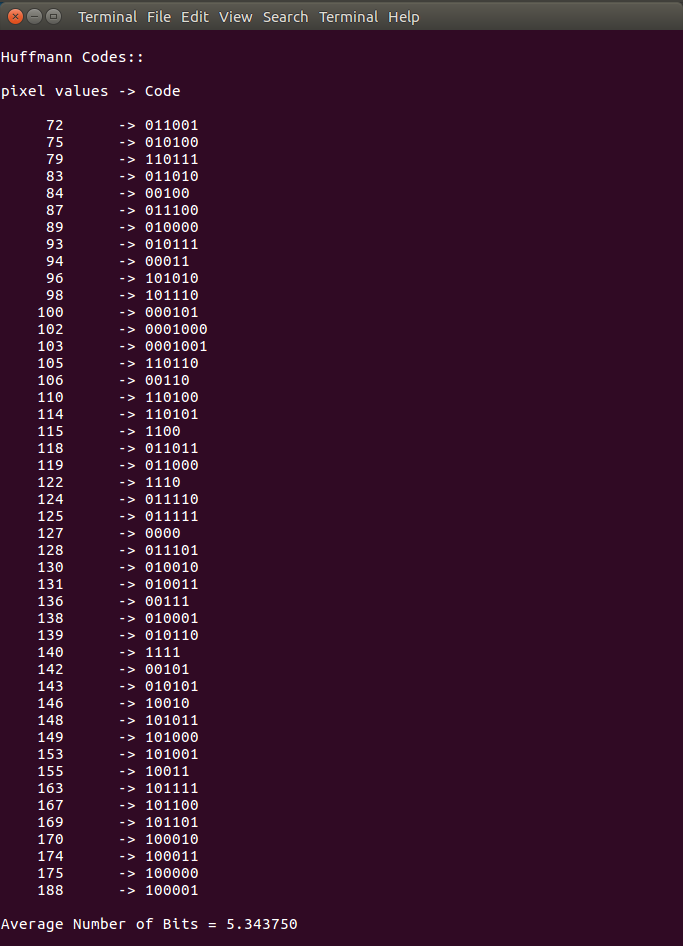Huffman Tree :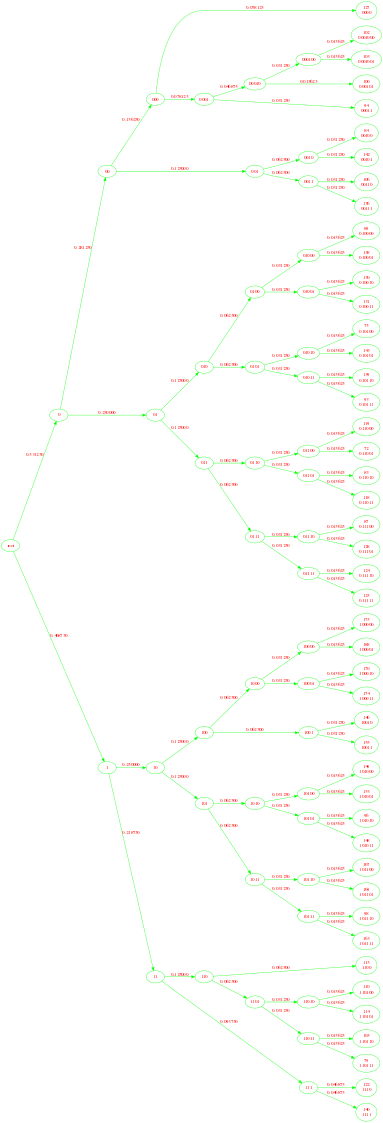My Personal Notes arrow_drop_upCheck out this Author's contributed articles.

If you like GeeksforGeeks and would like to contribute, you can also write an article using contribute.geeksforgeeks.org or mail your article to contribute@geeksforgeeks.org. See your article appearing on the GeeksforGeeks main page and help other Geeks.

Please Improve this article if you find anything incorrect by clicking on the "Improve Article" button below.

Improved By : nidhi_biet

Article Tags :

4

Please write to us at contribute@geeksforgeeks.org to report any issue with the above content.